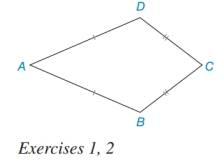Chapter 7.3, Problem 1EElementary Geometry For College St...

7th Edition
Alexander + 2 others
ISBN: 9781337614085

Solutions

Chapter
SectionElementary Geometry For College St...

7th Edition
Alexander + 2 others
ISBN: 9781337614085
Textbook Problem

Describe, if possible, how you would inscribe a circle within kite ABCD.To determine

To find:

The procedure to draw a circle inscribe a kite.

Explanation

Steps of construction to draw a circle inscribe a kite.

1) Construct the angle bisectors of BandC which intersect each other at O.

2) Draw a perpendicular from the centre O to side BC i.e., OM.

Still sussing out bartleby?

Check out a sample textbook solution.

See a sample solution

The Solution to Your Study Problems

Bartleby provides explanations to thousands of textbook problems written by our experts, many with advanced degrees!

Get Started

Write the sum in expanded form. 4. i=46i3

Single Variable Calculus: Early Transcendentals, Volume I

The circle with equation kx2 + ky2 = a2 lies inside the circle with equation x2 + y2 = a2, provided that k 1.

Applied Calculus for the Managerial, Life, and Social Sciences: A Brief Approach

Evaluate Evaluate the expression. 16. 250

Precalculus: Mathematics for Calculus (Standalone Book)

In problems 1-6, perform the indicated matrix operations with the following matrices.

Mathematical Applications for the Management, Life, and Social Sciences

Proof In Exercises 99-101, prove the differentiation formula. ddx[cothx]=csch2x

Calculus: Early Transcendental Functions (MindTap Course List)